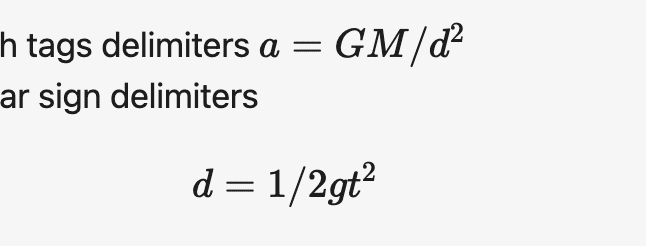# Entering capital and small letters in La Tex

• rb75
In summary, La Tex by default always displays the small letter in the form of a capital letter when writing equations if the letter is used in a different equation.f

#### rb75

When writing equations in La Tex, if I a enter a capital letter to represent a variable in the first equation I write, if I use that same letter in small form when writing a different equation, after examining the equation in preview mode, La Tex by default always displays the small letter in the form of a capital letter and vice versa. Is there a way to prevent La tex from doing this when writing equations?

This is not how LaTeX works …

$$E = mc^2$$

$$-1 = e^{i\pi}$$

That's not what I see. I get this (both in preview and as posted)*:

## X=\frac{A}{a} ##

## y=\frac{2A}{aX} ##

## z=xa^2 ##

* Win 10 & Chrome

Last edited:
I wrote the following equations:
The first was written using hash tag delimiters, and the second equation was written using dollar sign delimiters .
Could you please let me know if these equations have displayed properly in this post.

This equation was written using hash tags delimiters ##a = GM/d^2##
This equation was written using dollar sign delimiters $$d = 1/2gt^2$$
Thanks.

Screenshot:@rb75 If what you see is different from the screenshot posted above by Orodruin, please post a screenshot of what you are seeing so that we can understand the issue better.

The equations seem to be displayed properly.

When I view the equations in the my preview mode, the second equation is displayed improperly, the small letter g in the scond equation is displayed improperly in the form of a capital letter. Do you know if there's any way this can fixed so that the equation displays properly when I view it in my Preview mode?

Do you know if there's any way this can fixed so that the equation displays properly when I view it in my Preview mode?
Since nobody seems to be able to reproduce this, I'd guess nobody has a solution for it either.

@Wrichic Basu
Hi thanks for the help.
I would be glad to post you a screenshot Wrichic Basu, if you could please explain how I would do it. I'm new to PF and am very incompetent using the features in this editor. I've already been scolded once for copying and pasting text.

I sometimes have issues with the preview, different from yours, I think. I can always fix mine by just refreshing the page.

I would be glad to post you a screenshot Wrichic Basu, if you could please explain how I would do it.
Are you on your phone? If so , which kind?

If you are on a computer, what operating system version is it? If it's Win-10, you can use Windows-Shift-S to put it in Snapshot mode and drag the rectangle with your mouse to capture that screenshot.